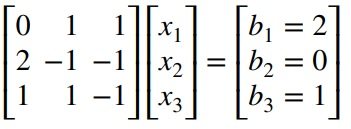Sale!

# MATLAB code of Gauss elimination

19

## Description

This MATLAB function implements the Gauss elimination algorithm solves

Ax=B

The sizes of matrices A, B are supposed to be mxm and mxn.

### For example :

Let us consider the following example:MATLAB code of Gauss elimination

The result will be :

x =

1.0000
1.0000
1.0000

## Reviews

There are no reviews yet.

SKU: GAUELMALG Category: